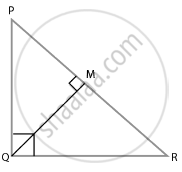# In a ∆PQR, PR2 – PQ2 = QR2 and M is a point on side PR such that QM ⊥ PR. Prove that QM2 = PM × MR. - Mathematics

Sum

In a ∆PQR, PR2 – PQ2 = QR2 and M is a point on side PR such that QM ⊥ PR. Prove that QM2 = PM × MR.

#### SolutionIn ∆PQR,

PR2 = QR2 and QM⊥PR

Using Pythagoras theorem, we have,

PR2 = PQ2 + QR2

∆PQR is right-angled triangle at Q.

From ∆QMR and ∆PMQ, we have,

∠M = ∠M

∠MQR = ∠QPM   ......[= 90°-∠R]

So, using the AAA similarity criteria,

We have,

∆QMR ∼ ∆PMQ

Also, we know that,

Area of triangles = 1/2 × base × height

So, by property of area of similar triangles,

⇒ (ar(∆QMR))/(Ar(PMQ)) = (QM)^2/(PM)^2

⇒ (ar(∆QMR))/(Ar(PMQ)) = (1/2 xx RM xx QM)/(1/2 xx PM xx QM)

⇒ (ar(∆QMR))/(ar(PMQ)) = (QM)^2/(PM)^2

QM2 = PM × RM

Hence proved.

Concept: Similarity of Triangles
Is there an error in this question or solution?

#### APPEARS IN

NCERT Mathematics Exemplar Class 10
Chapter 6 Triangles
Exercise 6.3 | Q 1 | Page 66

Share